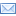SuperTeacherToolsCombining like terms and distributive property Speed Match Review Game

This game has been played 13 times
The best time ever was at 224 seconds.
The best time today is at seconds.
How To Play:
Drag the squares in the gray area and drop them in the matching squares in the blue area below.
If you drop a square in the correct spot, it will disappear.
If you make a mistake, the square will return to its original spot.
Good luck!

Let's Play Speed Match!

x = 4
x = 9
x = 10
-2 and 3
7x - 3
x = 8 and length = 11
-2z - 3
x = -2
7x + 4y - 20
10n + 3x - 2
3y + 2x - 1
x = 20
-3n + 15
Constant term
x - 6
x = -5
4x + 10
-11 + y - 2x
-9x - xy + 2
-5 and -6
x = -15
8w
Coefficient
Like terms
2x + 6
3(x + 2) = 18
The height of a triangle is 8. The base is x + 2. The area is 88. Solve for x.
-3(n - 5)
-4y + 2x + 7y - 1
Terms that have identical variable parts are called ______
The length of a rectangle is x + 3. The width is x + 5. The perimeter is 52. Solve for x.
2(x + 3)
7n - x + 4x + 3n - 2
2 - x(3 + y) - 6x
-5 + y - 6 + 3x - 5x
The area of a rectangle is 55. The length is x + 3. The width is 5. Solve for x and find the length.
2x + 4(x + y - 5) + x
-2(x - 4) - x = 23
The _____ of a term that has a variable is the number part of the term
5x + 2x - 3
Identify the constant terms: -5 + 4x - 2y - 6
3 + 5(x - 4) = 33
-2x + 3x - 4 - 2
Write and simplify an expression for the perimeter of a rectangle with length of x + 5 and width of x.
Identify the coefficients: -2x + 3x - 7
A rectangular sheet of plywood is three times longer than it is wide. Write and simplify an expression for the perimeter of the rectangle in terms of the width w.
2(x - 4) - 4(x + 2) = 18
2x - 3x + 2 = 4
A term that has a number, but no variable, is a ______
z - 3(z + 1)